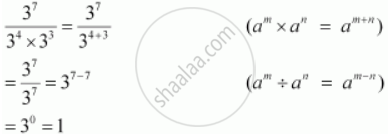Share

# Simplify and Express the Following in Exponential Form: 3exponents 7/(3exponents 4 Xx 3exponents 3) - CBSE Class 7 - Mathematics

ConceptLaws of Exponents Dividing Powers with the Same Base

#### Question

Simplify and express the following in exponential form:

3^7/(3^4 xx 3^3)

#### SolutionIs there an error in this question or solution?

#### APPEARS IN

NCERT Solution for Mathematics for Class 7 (2018 to Current)
Chapter 13: Exponents and Powers
Ex. 13.20 | Q: 2.05 | Page no. 260
Solution Simplify and Express the Following in Exponential Form: 3exponents 7/(3exponents 4 Xx 3exponents 3) Concept: Laws of Exponents - Dividing Powers with the Same Base.
S